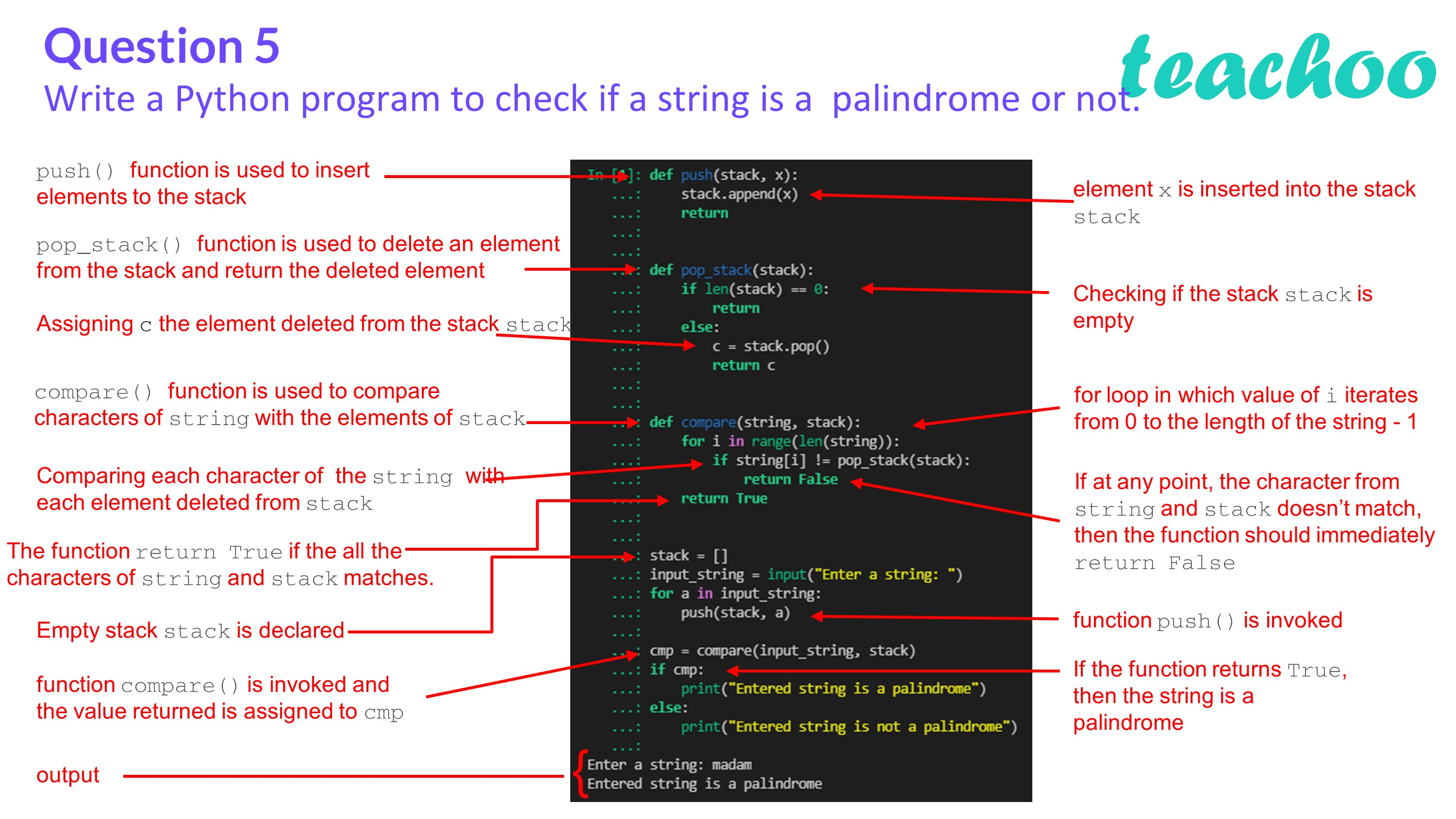Past Year - 2 Mark Questions

Computer Science - Class 12
Chapter 3 - Stacks

## Write a Python program to check if a string is a palindrome or not.

A palindrome is a sequence of characters that read the same backwards as forwards.

To check if a string is palindrome:

• First, insert all characters of the string into a stack.
• Then, using a for loop, keep popping the elements of the stack and compare them with corresponding characters of the string from the beginning.
• If at any point, there is a mismatch, then the string is not a palindrome.

``` Code: ```

``` def push(stack,x): ```

``` stack.append(x) ```

``` return ```

``` ```

``` def pop_stack(stack): ```

``` if len(stack)==0: ```

``` return ```

``` else: ```

``` c=stack.pop() ```

``` return c ```

``` def compare(string,stack): ```

``` for i in range(len(string)): ```

``` if string[i]!= pop_stack(stack): ```

``` return False ```

``` return True ```

``` ```

``` stack=[] ```

``` input_string=input("Enter a string: ") ```

``` for a in input_string: ```

``` push(stack,a) ```

``` ```

``` cmp=compare(input_string,stack) ```

``` if cmp: ```

``` print("Entered string is a palindrome") ```

``` else: ```

``` print("Entered string is not a palindrome") ```

Output:

``` Enter a string: madam ```

``` Entered string is a palindrome ```Learn in your speed, with individual attention - Teachoo Maths 1-on-1 Class

### Transcript

Write a Python program to check if a string is a palindrome or not. Answer: A palindrome is a sequence of characters that read the same backwards as forwards. Example: level, madam, refer, etc. To check if a string is palindrome: First, insert all characters of the string into a stack. Then, using a for loop, keep popping the elements of the stack and compare them with corresponding characters of the string from the beginning. If at any point, there is a mismatch, then the string is not a palindrome. Code: def push(stack,x): stack.append(x) return def pop_stack(stack): if len(stack)==0: return else: c=stack.pop() return c def compare(string,stack): for i in range(len(string)): if string[i]!= pop_stack(stack): return False return True stack=[] input_string=input("Enter a string: ") for a in input_string: push(stack,a) cmp=compare(input_string,stack) if cmp: print("Entered string is a palindrome") else: print("Entered string is not a palindrome") Output: Enter a string: madam Entered string is a palindrome